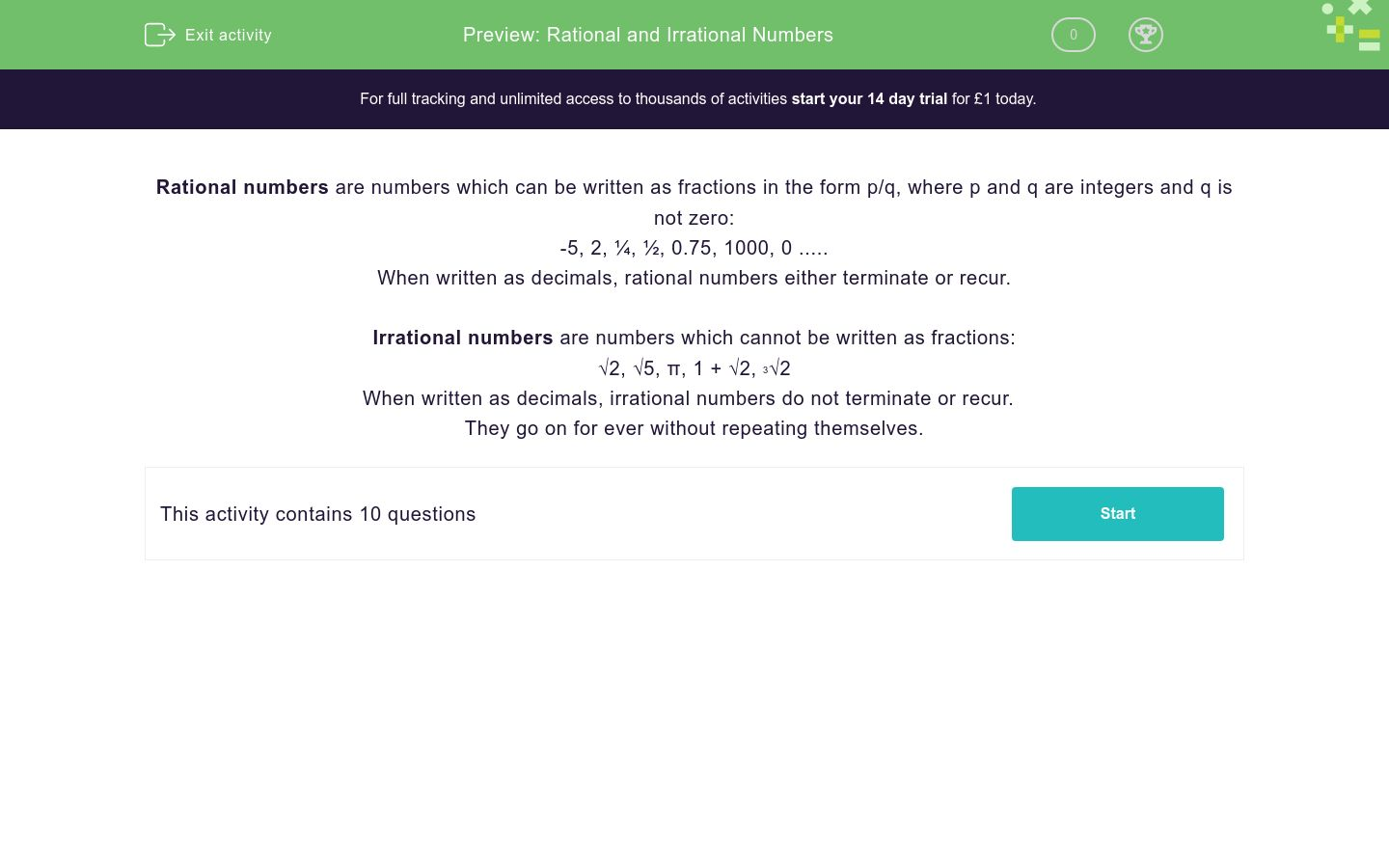# Rational and Irrational Numbers

In this worksheet, students learn to distinguish between rational and irrational numbers.Key stage:  KS 4

Curriculum topic:  Number

Curriculum subtopic:  Change Recurring Decimals into Their Corresponding Fractions and Vice Versa

Difficulty level:### QUESTION 1 of 10

Rational numbers are numbers which can be written as fractions in the form p/q, where p and q are integers and q is not zero:

-5, 2, ¼, ½, 0.75, 1000, 0 .....

When written as decimals, rational numbers either terminate or recur.

Irrational numbers are numbers which cannot be written as fractions:

√2, √5, π, 1 + √2, 3√2

When written as decimals, irrational numbers do not terminate or recur.

They go on for ever without repeating themselves.

Is the following number rational or irrational?

3

Rational

Irrational

Is the following number rational or irrational?

π

Rational

Irrational

Is the following number rational or irrational?

6

Rational

Irrational

Is the following number rational or irrational?

¾

Rational

Irrational

Is the following number rational or irrational?

Rational

Irrational

Is the following number rational or irrational?

√9

Rational

Irrational

Is the following number rational or irrational?

√10

Rational

Irrational

Is the following number rational or irrational?

5 - √12

Rational

Irrational

Is the following number rational or irrational?

3√12

Rational

Irrational

Is the following number rational or irrational?

3√1000

Rational

Irrational

• Question 1

Is the following number rational or irrational?

3

Irrational
EDDIE SAYS
Cannot be written as a fraction
• Question 2

Is the following number rational or irrational?

π

Irrational
EDDIE SAYS
π never recurs
• Question 3

Is the following number rational or irrational?

6

Rational
EDDIE SAYS
6 can be written as 6/1
• Question 4

Is the following number rational or irrational?

¾

Rational
EDDIE SAYS
¾ is a fraction and 0.75 terminates.
• Question 5

Is the following number rational or irrational?

Rational
EDDIE SAYS
6¾ can be written as 27/4
• Question 6

Is the following number rational or irrational?

√9

Rational
EDDIE SAYS
√9 = 3 which can be written as 3/1
• Question 7

Is the following number rational or irrational?

√10

Irrational
EDDIE SAYS
√10 is a non recurring decimal
• Question 8

Is the following number rational or irrational?

5 - √12

Irrational
EDDIE SAYS
√12 is a non recurring decimal
• Question 9

Is the following number rational or irrational?

3√12

Irrational
EDDIE SAYS
³√12 is a non recurring decimal
• Question 10

Is the following number rational or irrational?

3√1000

Rational
EDDIE SAYS
³√1000 = 10 which can be written as 10/1
---- OR ----

Sign up for a £1 trial so you can track and measure your child's progress on this activity.

### What is EdPlace?

We're your National Curriculum aligned online education content provider helping each child succeed in English, maths and science from year 1 to GCSE. With an EdPlace account you’ll be able to track and measure progress, helping each child achieve their best. We build confidence and attainment by personalising each child’s learning at a level that suits them.

Start your £1 trial